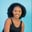Related Tags

numpy
python3

# How to use the np.multiply() function for a 2D array in PythonMaria Elijah

## Overview

The python library NumPy has a method called multiply() which can be used to multiply two input arrays.

### The numpy.multiply() method

The numpy.multiply() method returns the product of the two input arrays, element-wise.

Note: A two-dimensional (2D) array can be created using a list of lists in Python.

### Syntax

numpy.multiply(arr1, arr2, dtype=None, out=None)


### Parameters

• arr1: This represents the input data.
• arr2: This represents the input data.
• dtype: This is an optional parameter. It represents the return type of the array.
• out: This is an optional parameter. It represents the alternative output array in which the result is to be placed.

### Return value

The numpy.prod() method returns the product of the arr1 and arr2 arrays element by element.

Note: If the out parameter is specified, it returns an array reference to out.

### Example

The following code shows how to multiply two-dimensional (2D) arrays using the numpy.multipy() method.

# import numpy
import numpy as np
# create a two 2D arrays
arr1 = np.array([[2,6,5],[3,4,8]])
arr2 = np.array([[1,7,2],[10,9,4]])

# multiply the 2D arrays
# and store the result in arr_mul
arr_mul = np.multiply(arr1, arr2)

print(arr_mul)
Implementing the numpy.multiply fucntion

### Explanation

• Line 2: We import the numpy library.
• Lines 4–5: We create two 2D arrays, arr1 and arr2.
• Line 9: We use np.multiply() to multiple the arrays arr1 and arr2.
• Line 11: We display the result.

RELATED TAGS

numpy
python3

CONTRIBUTORMaria Elijah
RELATED COURSES

View all Courses

Keep Exploring

Learn in-demand tech skills in half the time